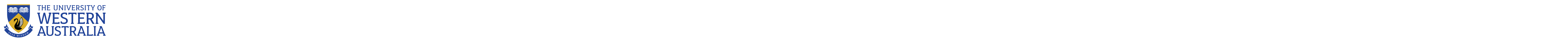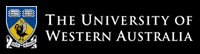School of Computer Science and Software Engineering

# School of Computer Science and Software Engineering

## CITS4406 Problem Solving and Programming

#### Labsheet 3: Simple Graphics Programming

This labsheet is in two parts: a number of review exercises taken from the text that reinforce concepts from the lectures; and an important problem-solving technique, motivated by its use in solving a larger-sized problem representative of the types of problems that can be solved with computers.

#### Review Exercises (Zelle, Chapter 3 & Chapter 4 upto 4.3)

1. Write a program that calculates the total cost of material required to make a sphere of certain radius. Prompt the user for radius in meters and material price per square meter.  Area of sphere = 4*pi*r*r

2. Write a program that prompts the user for the radius of a circle and then draws it using the graphics.py library. Hint: Put graphics.py in the same directory as your code and impot it.

3. Calculate the circumference and area of the circle and print it in the graphics window under the circle. Hint: use graphics.Text(graphics.Point(x,y), 'area').draw(win) where "win" is the name of your graphics window object.

4. Write a program that will draw a rectangle on a graphics window and move it horizontally.  Hints: use the graphics.Rectangle object and  the move method. Use time.sleep() to give a delay.

5. Try increasing and decreasing the speed of the rectangle.
6. Write a program to draw 20 concentric circles using a loop where the radius doubles each time. You will need to be careful of the size of the graphics window.

7. A local biologist needs a program to predict population growth. The inputs would be the initial number of organisms, the rate of growth (a real number greater than 0), the number of hours it takes to achieve this rate, and a number of hours during which the population grows. For example, one might start with a population of 500 organisms, a growth rate of 2, and a growth period to achieve this rate of 6 hours. Assuming that none of the organisms die, this would imply that this population would double in size every 6 hours. Thus after 6 hours, we would have 1000 organisms, and after 12 hours, we would have 2000 organisms. Write a program that takes these inputs and displays a prediction of the total population.

8.An investor wants to know how many years will it take for his money to double given an interest rate. Write a function that takes the interest rate as a parameter and prints the number of years required for an investment to double.

9.The greatest common divisor (GCD) of two positive integers is the largest integer that evenly divides into both of them. For example, the GCD of 18 and 12 is 6. Euclid's algorithm to calculate GCDs is as follows:

• Compute the remainder of dividing the larger number by the smaller number.
• Replace the larger number with the smaller number and the smaller number with the remainder.
• Repeat this process until the smaller number is zero: at this point the larger number is the GCD.
Write a program that lets the user enter two integers and that prints each step in the process of using the Euclidean algorithm to find their GCD.

10.Assume b represents an unsigned binary number of arbitrary length. Write a function to calculate the decimal equivalent of b. Pass the binary number b as a parameter of type str to the function. For example, if b = '00000100', its decimal equivalent is 4.

Try your algorithm out by entering each step into Python and make sure it works. What decimal value do you get for the binary number 00111100?

#### Problem Solving Technique: Reduction and Analogy

Reduction and Analogy are general problem solving techniques. Suppose that we are presented with an unfamiliar problem but we notice that there is some relationship between it and some other problem that we do know how to solve. If you can "reduce" the new problem to the old one, or see that they are alike, or analagous, and may have similar solutions then you may be able to solve the new one by making use of, or modifying, the solution to the known problem.

See if you can use reduction or analogy to solve the following problems.

Example problem 1:

Write a code segment that prints half of an n x n square, e.g.:

#####
####
###
##
#

Now extend this program to print a sideways triangle:

#
##
###
####
#####
####
###
##
#

Example problem 2:

We are conducting an experiment in improving rice field yields and have enlisted the help of many small scale farmers across the Chiba prefecture, Japan. We are varying eight factors that affect the rice production (per hectare) and each farmer will tell us the way that he (in two cases, she) treated the rice as it grew when he delivers the rice to the refinery.

The eight variables/questions are as follows:

• Did you use extra nitrogen-based fertiliser? (y/n)
• Did you use extra phosphate-based fertiliser? (y/n)
• Did you allow early flooding of the field? (y/n)
• Was the field left fallow (empty) the previous season? (y/n)
• Did you harvest early? (y/n)
• Did you drain the field before harvest? (y/n)
• Were the grains dried in the sun before delivery? (y/n)
• Did you use the more expensive new variety? (y/n)

Note that there are thus 256 different ways that any given field of rice can be treated, depending on the answers to those questions.

Upon delivery, each truckful of rice will have information recorded and the refinery staff will be given a piece of paper with a unique delivery sequence number and a treatment number.

Your task is to write a Python program that asks the eight questions in order, to be asked to the farmer on delivery, and printout one treatment number 0 to 255, that summarises how his rice was treated.

You can choose the way the treatment numbers work but two fields of rice that get the same treatment, on all eight questions, should get the same treatment number. Two fields that are different on any question need to get different numbers. The number should range from 0 to 255.

Extended Challenge: Write a program to turn your treatment numbers into answers for the eight questions.

# The University of Western Australia

## University information

CRICOS Code: 00126G